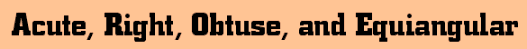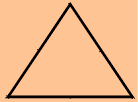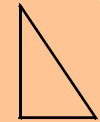Triangles are structurally strong and often used in the construction of bridges and buildings.Traingles can be classified by their angles. An Acute Triangle has all angles less than 90 degrees.An Obtuse Triangle has one angle greater than 90 degrees.A Right Triangle has one 90 degree angle.An Equiangular Triangle has all 3 angles equal to 60 degreesClassify this triangle by its angles right acute equiangular obtuse
 Classify this triangle by its angles right acute equiangular obtuseClassify this triangle by its angles right acute equiangular obtuse
 Classify this triangle by its angles right acute equiangular obtuse